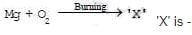# Important Questions (1 mark): Atoms and Molecules

## 20 Questions MCQ Test NCERT Textbooks (Class 6 to Class 12) | Important Questions (1 mark): Atoms and Molecules

Description
Attempt Important Questions (1 mark): Atoms and Molecules | 20 questions in 25 minutes | Mock test for UPSC preparation | Free important questions MCQ to study NCERT Textbooks (Class 6 to Class 12) for UPSC Exam | Download free PDF with solutions
QUESTION: 1

### The elements present in baking soda are -

Solution: Baking soda, also called sodium bicarbonate, is a common baking ingredient, cleaner, deodorizer and pH regulator. It is commonly sold as a white powder that looks similar to baking powder. Unlike baking powder, which contains acidic ingredients, however, baking soda is a single compound composed of only four elements: sodium, hydrogen, carbon and oxygen.
QUESTION: 2

### The first scientist to use of the symbols of elements was-

Solution:
John Dalton
John Dalton was the first scientist to use symbols for elements. He was first to use chemical symbols for elements perfectly in his table of elements , which made chemical chemistry easy.
QUESTION: 3

### The overall charge on an ionic compound is equal to -

Solution:

The compound made up of ions is known as ionic compound. Since an ionic compound consists of equal number of positive and negative ions, the overall charge of an ionic compound is zero.

QUESTION: 4

The chemical formula of the copper nitrate -

Solution: Copper nitrate, also known as Cupric nitrate and its chemical formula is Cu(NO3)2
QUESTION: 5

The number of carbon atoms in 1g of CaCO3 is-

Solution:

First we calculate the number of moles of CaCO3
Molecular weight of CaCO3 = 100g
Therefore, 100g of CaCO3 will have Avogadro number of molecules (6.022x10²³).
so 1g will have
(6.022x10²³) x 1/100
= 6.022x10²¹
Now one molecule of CaCO3 has 1 carbon atom. So, 6.022x10²¹ molecules will have 6.022x10²¹ atoms of Carbon.

QUESTION: 6

The mass of a single atom of carbon is -

Solution:

Mass of one atom = Mass of one mole of atom / Avogadro’s no.
=  12/  6.02 x 10²³
Mass of one carbon atom = 1.994 x 10¯²³

QUESTION: 7

The mass of 1 u is -

Solution:

1u = 1/12 Mass of the carbon atom (12c)
6.022X1023 atoms of carbon = 12 g
I atom of carbon = 12/6.022x1023 g
= 1.99x10-23 g
1u = 1.99 x 10−23 /12
= 1.66x10-24 g

QUESTION: 8

How many molecules are present in 9g of water -

Solution:
No. of molecules in 9g of water:

Molecular mass of water(H2O)= (1x2)+16=18g/mol

1 mole of water=18g of water contains=6.022x10^23 molecules of water

then 9g of water will have= (6.022x10^23/18)x9 = 3.011x10^23 molecules
QUESTION: 9Solution:
QUESTION: 10

The formula of sulphuric acid is-

Solution:
QUESTION: 11

Which of the following has largest number of particles?

Solution:

The largest number of particles are in (d) 2g of H2
8g of CH4 = 8 / 16 = 0.5 moles = 3.011 × 10²³ atoms
4.4g of CO2 = 4.4 / 44 = 0.1 moles = 0.6022 × 10²³ atoms
34.2g of C12H22O11 = 34.2 / 342 = 0.1 moles = 0.6022 × 10²³ atoms
2g of H2 = 2 / 2 = 1 moles = 6.022 × 10²³ atoms
Hence, the correct answer is Option D.

QUESTION: 12

Mass of one Avogadro's number of O atom is equal to -

Solution: 1 mole of any atom is = 6.022 x 10 ^23 atom ( avogadro's number ) now, there are Avogadro's number of O atom, which will be = 1 mole ie ; we have 1 mole oxygen atom . mole = weight / molecular weight 1 = weight / 16 ( molecular weight of O = 16 a.m.u ) weight = 16 g ( answer )
QUESTION: 13

How many molecules are present in one gram of hydrogen gas?

Solution:

The correct option is B.
One gram of hydrogen contains 1 mole of hydrogen as its atomic weight is 1
1 mole =6.023×1023 atoms.

But, hydrogen is a diatomic molecule.
So, its atoms are found in pairs.

Amount of molecule =3.02×1023

∴3.02×1023
molecules are present in one gram of hydrogen.

QUESTION: 14

The correct formula of aluminium sulphate is

Solution:
QUESTION: 15

Which of the following has highest intermolecular forces of attraction -

Solution: As the carbon dioxide is in solid form(dry ice) so it has gained all the properties of solid i.e. now they have highest intermolecular force between them.
QUESTION: 16

Which of the following is not correct regarding gases?

Solution:

Gases have strong tendency to diffuse. Gases have high kinetic energy which because of weak intermolecular forces. This makes them move faster which makes their diffusion fast.

QUESTION: 17

The Boiling point of water at normal atmospheric pressure is -

Solution:

The correct option is B.
Temperature scale that registers the freezing point of water as 0 degrees C and the boiling point as 100 degrees C under normal atmospheric pressure. Temperature scale in which zero occurs at absolute zero and each degree equals one kelvin. Water freezes at 273.15 K and boils at 373.15 K.

QUESTION: 18

Avogadro's number represents the number, of atoms in-

Solution:
QUESTION: 19

Molecular mass of ozone is

Solution:
QUESTION: 20

Which of the following is not correct according to Dalton's atomic theory?

Solution:

The correct option is B.
According to Dalton's atomic theory, all matter, whether an element, a compound or a mixture is composed of small particles called atoms. ... Atoms are indivisible particles, which cannot be created or destroyed in a chemical reaction. Atoms of a given element are identical in mass and chemical properties.Use Code STAYHOME200 and get INR 200 additional OFF Use Coupon Code